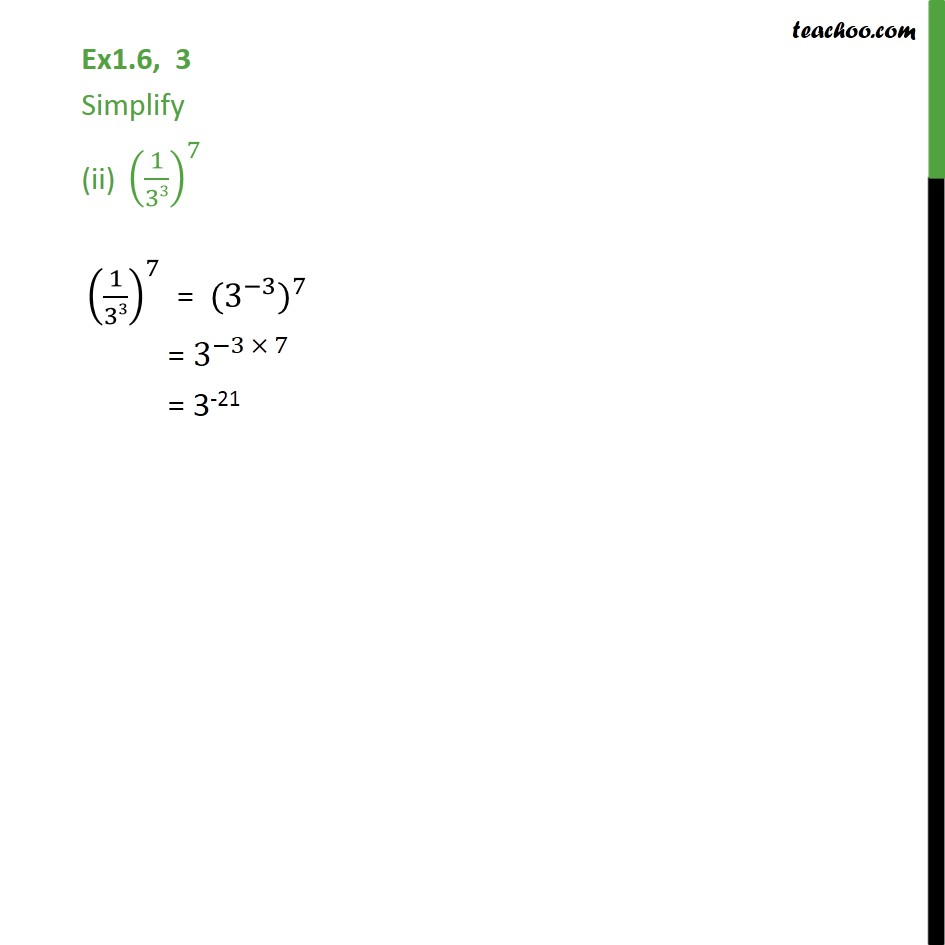1. Chapter 1 Class 9 Number Systems (Term 1)
2. Serial order wise
3. Ex 1.6

Transcript

Ex1.6, 3 Simplify (ii) (1/33)^7 (1/33)^7 = (3^( 3)) ^7 = 3^( 3 7) = 3-21